# Determinant - Formulas Notes | EduRev

## JEE : Determinant - Formulas Notes | EduRev

The document Determinant - Formulas Notes | EduRev is a part of the JEE Course Mathematics For JEE.
All you need of JEE at this link: JEE

1.The symbol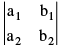is called the determinant of order two.
It's value is given by  : D = a1 b2 − a2 b1

2.The symbol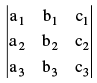is called the determinant of order three.
Its value can be found as : D =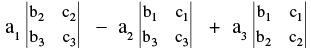Or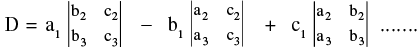and so on. In this manner we can expand a determinant in 6 ways using elements of  ; R1, R2, R3  or  C1, C2, C3.

3. Following examples of short hand writing large expressions are :
(i) The lines : a1x + b1y + c1 = 0........ (1)
a2x + b2y + c2 = 0........ (2)
a3x + b3y + c3 = 0........ (3)
are concurrent if,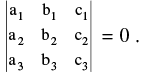Condition for the consistency of  three  simultaneous linear equations  in 2 variables.
(ii) ax² + 2 hxy + by² + 2 gx + 2 fy + c = 0 represents a pair of straight lines if abc + 2 fgh − af² − bg² − ch² = 0 =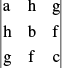(iii) Area of a  triangle whose vertices are  (xr, yr) ;  r = 1 , 2 , 3  is  :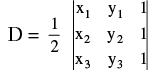If  D = 0 then the three points are collinear.
(iv) Equation of a straight line passing through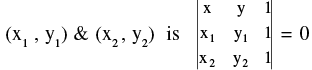4. MINORS  : The minor of a given element of a determinant is the determinant of the elements which remain after deleting the row & the column in which the given element stands For example, the minor of a1 in (Key Concept 2)  is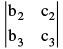&  the minor of b2 is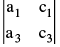. Hence a determinant of order two will have “4 minors” & a determinant of order  three will have “9 minors” .

5. COFACTOR : If Mij represents the minor of some typical element then the cofactor is defined as  :
Cij = (−1)i+j . Mij  ;  Where i & j denotes the row & column in which the particular element  lies. Note that the value of a determinant of order three in terms of ‘Minor’ & ‘Cofactor’ can be written as :  D = a11M11 − a12M12 + a13M13 OR  D = a11C11 + a12C12 + a13C13  & so on .......

6. PROPERTIES  OF  DETERMINANTS :
P−1 : The value of a determinant remains unaltered, if the rows & columns are inter changed. e.g. if D =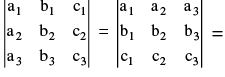D′ D & D′ are transpose of each other.  If D′ = − D then it is SKEW SYMMETRIC determinant but D′ = D ⇒ 2 D = 0 ⇒ D = 0 ⇒ Skew  symmetric determinant of third order  has the value zero.
P−2 : If any two rows (or  columns) of a determinant be interchanged, the value of determinant is changed in sign only. e.g. Let: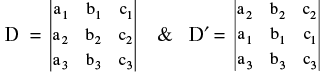Then D′ = − D.
P−3 : If a determinant has any two rows (or columns) identical, then its value is zero. e.g. Let D =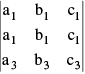then it can be verified that D = 0.
P−4 : If all the elements of any row (or column) be multiplied by the same number, then the determinant is multiplied by that number.
e.g. If D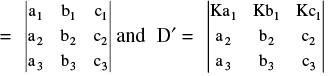Then D′= KD
P−5 : If each element of any row (or column) can be expressed as a sum of two terms then the determinant can be expressed as the sum of two determinants. e.g.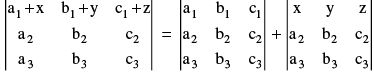P−6 : The value of a determinant is not altered by adding to the elements of any row (or column) the same multiples of the corresponding elements of any other row (or column).e.g. Let D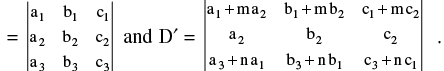Then  D′ = D.
Note : that while applying this property ATLEAST ONE ROW (OR COLUMN) must remain unchanged.
P−7 : If by putting  x = a the value of a determinant vanishes then (x − a) is a  factor of the determinant.

7. MULTIPLICATION OF TWO DETERMINANTS : (i)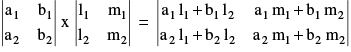Similarly two determinants of order three are multiplied.
(ii) If D =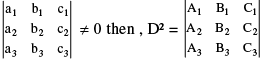where Ai, Bi, Ci are cofactors
PROOF : Consider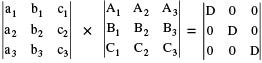Note : a1A2 + b1B2 + c1C2 = 0 etc. therefore,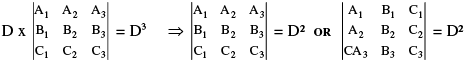8. SYSTEM OF LINEAR EQUATION  (IN TWO VARIABLES) :
(i) Consistent Equations : Definite & unique solution.  [intersecting lines]
(ii) Inconsistent Equation : No solution. [Parallel line]
(iii) Dependent equation : Infinite solutions.  [Identical lines]
Let  a1x + b1y + c1 = 0  & a2x + b2y + c2 = 0 then :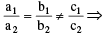Given equations are inconsistent &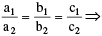Given equations are dependent

9. CRAMER'S  RULE : [ SIMULTANEOUS EQUATIONS INVOLVING THREE UNKNOWNS]
Let, a1x + b1y + c1z = d1 ...(I) ; a2x + b2y + c2z = d2 ... (II) ; a3x + b3y + c3z = d3 ... (III)
Then,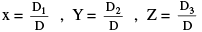.
Where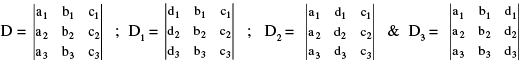NOTE : (a) If  D ≠ 0 and alteast one of D1, D2, D3 ≠ 0, then the given system of equations are consistent and have unique non trivial solution.
(b) If  D ≠ 0  &  D1 = D2 = D3 = 0,  then the given system of equations are consistent and have trivial solution only.
(c) If D = D1 = D2 = D= 0, then the given system of equations are consistentand have infinite solutions. In case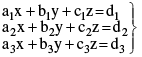represents these parallel planes then also D = D1 = D2 = D3 = 0 but the system is inconsistent.
(d) If D = 0 but at least one of D1, D2, D3 is not zero then the equations are in consistent and have no solution.

10. If x, y, z  are not all zero, the condition for a1x + b1y + c1z = 0  ;  a2x + b2y + c2z = 0  & a3x + b3y + c3z = 0 to be consistent in x, y, z is that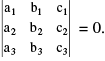Remember that if a  given system of linear equations have Only Zero Solution for all its variables then the given equations are said to have TRIVIAL SOLUTION.

Offer running on EduRev: Apply code STAYHOME200 to get INR 200 off on our premium plan EduRev Infinity!

## Mathematics For JEE

353 videos|269 docs|272 tests

,

,

,

,

,

,

,

,

,

,

,

,

,

,

,

,

,

,

,

,

,

;